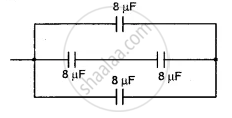# You Are Provided with 8 μF Capacitors. Show with the Help of a Diagram How You Will Arrange Minimum Number of Them to Get a Resultant Capacitance of 20 μF. - Physics (Theory)

Short Note

You are provided with 8 μF capacitors. Show with the help of a diagram how you will arrange minimum number of them to get a resultant capacitance of 20 μF.

#### Solution

The arrangement of 8 pF capacitor to get a capacity of 20 μF is as shown in the fig. Total capacity = 8 + 4 + 8 = 20 μFConcept: Capacitors and Capacitance
Is there an error in this question or solution?